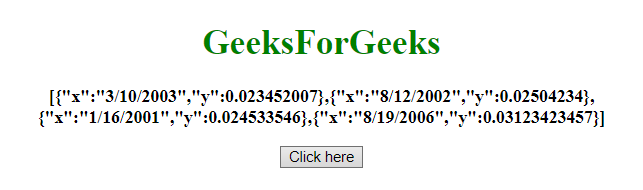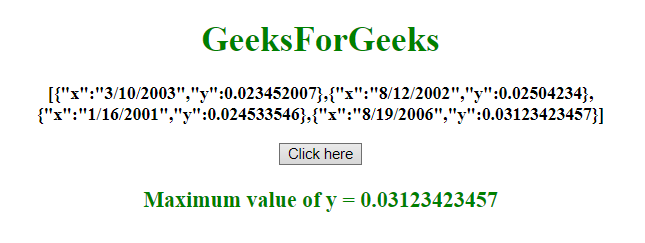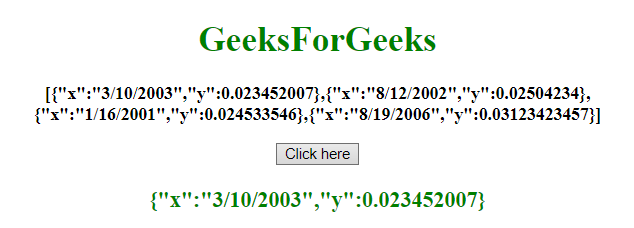# Max/Min value of an attribute in an array of objects in JavaScript

Given an array of objects and the task is to get the maximum and minimum value from the array of objects. There are a few methods used which are discussed below:

• Javascript apply() method: It is write method which is used on different objects. This method is different from the function call() because of taking arguments as an array.

Syntax:

`apply()`

Return value: It returns the method values of a given function.

• JavaScript Array map() Method: This method is used to create a new array with the results of calling a function for each element of array. This method calls the given function once for each element in an array, in order.

Syntax:

`array.map(function(cValue, index, arr), thisValue)`

Parameters:

• function(cValue, index, arr): It is required parameter. It is a function to be run for each element in the array.
• cValue: It is required parameter. It specifies the value of the current element.
• index: It is optional parameter. It specifies the array index of the current element.
• arr: It is optional parameter. It specifies the array object of the current element belongs to.
• thisValue: It is optional parameter. It specifies the value to be passed to the function to be used as its “this” value. If not used, the value “undefined” will be passed as its “this” value.

Return value: It returns an array having the results of calling the provided function for every element of the original array.

• JavaScript Array reduce() Method: This method reduces the array to a single value. This method runs a defined function for every value of the array (from left-to-right). The return value of the function is stored in an accumulator (result or total).

Syntax:

```array.reduce(function(total, curValue, curIndex, arr), initialValue)
```

Parameters:

• function(total, curValue, curIndex, arr): It is required parameter. It is a function to be run for each element in the array.
• total: It is required parameter. It specifies the initialValue, or the previously returned value of the function.
• curValue: It is required parameter. It specifies the value of the current element.
• curIndex: It is required parameter. It specifies the array index of current element.
• arr: It is optional parameter. It specifies the array object the current element belongs to.
• initialValue: It is optional parameter. It specifies the value to be passed to the function as the initial value.

Return value: It returns the accumulated result from the last call of the callback function.

Example 1: This example gets the maximum value of y property by using apply() and map() method.

 `  ` `<``html``>  ` `    ``<``head``>  ` `        ``<``title``>  ` `            ``Max value of an attribute in an array of objects ` `        `` ` `    ``  ` `     `  `    ``<``body` `style` `= ``"text-align:center;"``>  ` `     `  `        ``<``h1` `style` `= ``"color:green;"` `>  ` `            ``GeeksForGeeks  ` `        ``  ` `     `  `        ``<``p` `id` `= ``"GFG_UP"` `style` `= ``"font-size: 16px; font-weight: bold;"``> ` `        `` ` `         `  `        ``<``button` `onclick` `= ``"gfg_Run()"``>  ` `            ``Click here ` `        `` ` `         `  `        ``<``p` `id` `= ``"GFG_DOWN"` `style` `=  ` `            ``"color:green; font-size: 20px; font-weight: bold;"``> ` `        `` ` `         `  `        ``<``script``> ` `            ``var el_up = document.getElementById("GFG_UP"); ` `            ``var el_down = document.getElementById("GFG_DOWN"); ` `             `  `            ``var array = [ ` `                ``{ "x": "3/10/2003", "y": 0.023452007 },  ` `                ``{ "x": "8/12/2002", "y": 0.02504234 },  ` `                ``{ "x": "1/16/2001", "y": 0.024533546 }, ` `                ``{ "x": "8/19/2006", "y": 0.03123423457 }]; ` `                 `  `            ``el_up.innerHTML = JSON.stringify(array); ` `             `  `            ``function gfg_Run() { ` `                ``el_down.innerHTML = "Maximum value of y = " +  ` `                ``Math.max.apply(Math, array.map(function(o) { ` `                    ``return o.y;  ` `                ``})); ` `            ``}          ` `        ``  ` `    ``  ` `                     `

Output:

• Before clicking on the button:• After clicking on the button:Example 2: This example gets the minimum value of y property by using array.reduce() method. It returns the whole object.

 `  ` `<``html``>  ` `    ``<``head``>  ` `        ``<``title``>  ` `            ``Min value of an attribute in an array of objects ` `        `` ` `    ``  ` ` `  `    ``<``body` `style` `= ``"text-align:center;"``>  ` `         `  `        ``<``h1` `style` `= ``"color:green;"` `>  ` `            ``GeeksForGeeks  ` `        ``  ` `         `  `        ``<``p` `id` `= ``"GFG_UP"` `style` `= ``"font-size: 16px; font-weight: bold;"``> ` `        `` ` `         `  `        ``<``button` `onclick` `= ``"gfg_Run()"``>  ` `            ``Click here ` `        `` ` `         `  `        ``<``p` `id` `= ``"GFG_DOWN"` `style` `=  ` `            ``"color:green; font-size: 20px; font-weight: bold;"``> ` `        `` ` `         `  `        ``<``script``> ` `            ``var el_up = document.getElementById("GFG_UP"); ` `            ``var el_down = document.getElementById("GFG_DOWN"); ` `             `  `            ``var array = [ ` `                ``{ "x": "3/10/2003", "y": 0.023452007 },  ` `                ``{ "x": "8/12/2002", "y": 0.02504234 },  ` `                ``{ "x": "1/16/2001", "y": 0.024533546 },  ` `                ``{ "x": "8/19/2006", "y": 0.03123423457 }]; ` `                 `  `            ``el_up.innerHTML = JSON.stringify(array); ` `             `  `            ``function gfg_Run() { ` `                ``el_down.innerHTML =  ` `                ``JSON.stringify(array.reduce(function(prev, current) { ` `                    ``return (prev.y < ``current.y``) ? prev : current ` `                ``})); ` `            ``}          ` `        ``  ` `    ``  ` `                     `

Output:

• Before clicking on the button:• After clicking on the button:My Personal Notes arrow_drop_upCheck out this Author's contributed articles.

If you like GeeksforGeeks and would like to contribute, you can also write an article using contribute.geeksforgeeks.org or mail your article to contribute@geeksforgeeks.org. See your article appearing on the GeeksforGeeks main page and help other Geeks.

Please Improve this article if you find anything incorrect by clicking on the "Improve Article" button below.